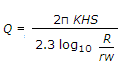# Civil Engineering - Water Supply Engineering - Discussion

### Discussion :: Water Supply Engineering - Section 4 (Q.No.8)

8.

The dischargefor the confined tube well is obtained from

 [A]. Thiem's formula [B]. Darcy's formula [C]. Tolman's formula [D]. Dupuits formula.

Explanation:

No answer description available for this question.

 Praveen said: (Jul 31, 2016) A) Thiem's formula is the correct answer.

 Vikas Chauhan said: (Feb 24, 2017) You are right @Praveen. Because Dupuits formula is for unconfined tube wells.

 Pritamdas said: (Mar 12, 2017) Dupit is correct. I agree.

 Roy said: (Aug 26, 2017) Yes, A is the correct answer.

 Garry said: (Oct 3, 2017) This equation for confined well obtained from steady flow using darcy equation and the equation achieved is called Thieme's equation.

 Garry said: (Oct 3, 2017) The correct answer is B.

 Roop Kumar said: (Mar 14, 2018) Dupits for unconfined aquifer.

 Satish Verma said: (Apr 18, 2018) Option A is right.

 Heer said: (Sep 25, 2019) Given answer is correct one because in question it is given R and Rw. Which is considered in Dupuit's confined aquifer equation. In Dupuit's method, the integration limit is considered is R and Rw. Where R = radius of influence. Rw = radius of the main pumped well. While in Thiem's formula for confined aquifers it is given r2 and r1, In Thiem's formula the integration limit is considered is r2 and r1. Where r2 and r2 = radii of two observation wells.

 Heer said: (Sep 25, 2019) r2 and r1 = radii of two observeration wells. In Thiem's formula. And In Dupuit's formula, R = radius of influence, Rw = radius of the main pumped well. So, the given answer is correct.